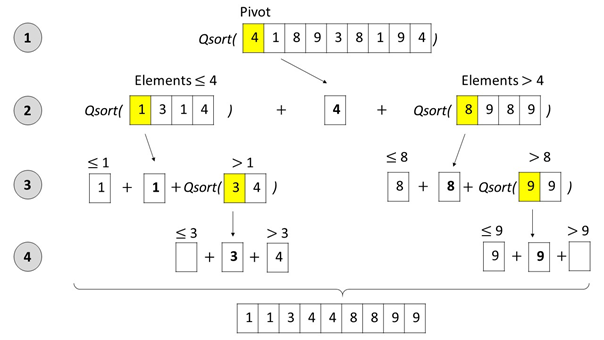# The Quicksort Algorithm in Python

Rate this post

For a coder, understanding the Quicksort algorithm is like knowing the secret of 42—either you get it, or you don’t belong to the club. If you don’t know either, let’s work on the first one!

## The Quicksort Algorithm — A Python Implementation

```def qsort(L):

# The empty list is sorted by default
if L == []:
return []

# Pivot is first element
pivot = L[0:1]

# Sort elements that are smaller than pivot
left = qsort([x for x in L[1:] if x < L])

# Sort elements that are larger or equal than pivot
right = qsort([x for x in L[1:] if x >= L])

# Concatenate the three parts to the sorted list
# assuming left and right are already sorted recursively
return left + pivot + right

print(qsort([0,33,22]))
```

The algorithm is a variant of the popular Quicksort algorithm. The function `qsort` sorts the list. But why?

## Quicksort Explanation

Quicksort selects a pivot element from the list. In the code snippet, it selects the first element of the list using indexing, i.e., `L`.

• Then, the algorithm moves all elements that are smaller than the pivot to the left side.
• Similarly, it moves elements that are larger or equal than the pivot to the right side.

This is repeated in a recursive manner for the left and the right lists.

Suppose, you create a new list as follows. You put all elements that are smaller than the pivot on the left, then the pivot, then all elements that are larger or equal the pivot on the right. The resulting list feels a bit more sorted, right? If the two sublists were already sorted, the list would be perfectly sorted. This is where the recursive call of `qsort` comes into play. It takes over the problem of sorting each sublist by applying the same scheme of pivoting and recursion to the sublist.

Here’s a visual explanation, I prepared as part of my book Python One-Liners:Figure: The Quicksort algorithm selects a pivot element, splits up the list into (i) an unsorted sublist with all elements that are smaller or equal than the pivot, and (ii) an unsorted sublist with all elements that are larger than the pivot. Next, the Quicksort algorithm is called recursively on the two unsorted sublists to sort them. As soon as the sublists contain maximally one element, they are sorted by definition – the recursion ends. At every recursion level, the three sublists (left, pivot, right) are concatenated before the resulting list is handed to the higher recursion level.

## Interactive Interpreter Quicksort

You can test this code in our interactive code shell:

Exercise: Try sorting a string list—does it work?

## Code Puzzle Quicksort

Here’s a code puzzle regarding the Quicksort algorithm that you may enjoy—click the image to solve it in our interactive Finxter puzzle app:

Are you a master coder?